# Behavioral Science - Epidemiology / Biostatistics Flashcards Preview

## First Aid > Behavioral Science - Epidemiology / Biostatistics > Flashcards

Flashcards in Behavioral Science - Epidemiology / Biostatistics Deck (52):
1

2

3

4

5

6

7

8

9

10

11

## Evaluation of diagnostic tests

### Uses 2 × 2 table comparing test results with the actual presence of disease. TP = true positive FP = false positive TN = true negative FN = false negative Sensitivity and specificity are fixed properties of a test (vs. PPV and NPV).12

13

14

15

## Negative predictive value (NPV) (51)

### Definition Proportion of negative test results that are true negative. Probability that person actually is disease free given a negative test result. NPV varies inversely with prevalence or pretest probability High pretest probability --> low NPV Equation = TN / (FN + TN)16

17

## Odds ratio (OR) Definition Equations

### Definition Typically used in case-control studies. Odds that the group with the disease (cases) was exposed to a risk factor (a/c) divided by the odds that the group without the disease (controls) was exposed (b/d). Equations OR = (a/c) / (b/d) = ad / bc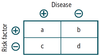18

## Relative risk (RR) Definition Equations

### Definition Typically used in cohort studies. Risk of developing disease in the exposed group divided by risk in the unexposed group e.g., if 21% of smokers develop lung cancer vs. 1% of nonsmokers, RR = 21/1 = 21 If prevalence is low, RR ≈ OR. Equations RR = [ a / (a+b) ] / [ c / (c+d) ]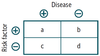19

20

## Attributable risk (AR) Definition Equations

### Definition The difference in risk between exposed and unexposed groups, or the proportion of disease occurrences that are attributable to the exposure e.g., if risk of lung cancer in smokers is 21% and risk in nonsmokers is 1%, then 20% (or .20) of the 21% risk of lung cancer in smokers is attributable to smoking. Equations AR = [ a / (a+b) ] - [ c / (c+d) ]21

## Absolute risk reduction (ARR) Definition Equations

### Definition The difference in risk (not the proportion) attributable to the intervention as compared to a control e.g., if 8% of people who receive a placebo vaccine develop flu vs. 2% of people who receive a flu vaccine, then ARR = 8% - 2% = 6% = .06. Equations ARR = [ c / (c+d) ] - [ a / (a+b) ]22

23

24

25

## Accuracy

### The trueness of test measurements (validity). The absence of systematic error or bias in a test. Systematic error—reduces accuracy in a test.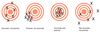26

27

28

29

30

31

32

33

34

35

## Normal distribution

### Gaussian, also called bell-shaped. Mean = median = mode.36

## Bimodal distribution

### Suggests two different populations e.g., metabolic polymorphism such as fast vs. slow acetylators; suicide rate by age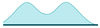37

## Positive skew

### Typically, mean > median > mode. Asymmetry with longer tail on right.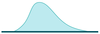38

## Negative skew

### Typically, mean < median < mode. Asymmetry with longer tail on left.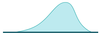39

## Null Hypothesis (H0)

### Hypothesis of no difference e.g., there is no association between the disease and the risk factor in the population40

## Alternative Hypothesis (H1)

### Hypothesis of some difference e.g., there is some association between the disease and the risk factor in the population41

## Table: Power, Type 1 Error, Type 2 Error, and Correct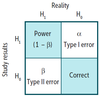42

43

44

45

46

47

48

49

50

51

52